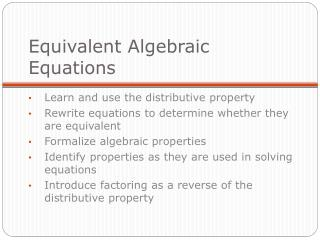DownloadDownload PresentationEquivalent Algebraic Equations

# Equivalent Algebraic Equations

Download Presentation## Equivalent Algebraic Equations

- - - - - - - - - - - - - - - - - - - - - - - - - - - E N D - - - - - - - - - - - - - - - - - - - - - - - - - - -
##### Presentation Transcript

1. Equivalent Algebraic Equations Learn and use the distributive property Rewrite equations to determine whether they are equivalent Formalize algebraic properties Identify properties as they are used in solving equations Introduce factoring as a reverse of the distributive property

2. In the previous lesson you learned to write the equation of line using the point-slope form. You were given the slope and a point. • But remember that a line goes through many points. Will the equation be equivalent if it written using another point? • In this lesson you will learn how to identify different equations that describe the same line.

3. If a line with slope 2 that passes through the point (-4,3) can be described by the equation y = 3 + 2(x+4). • This line also passes through (1, 13), so it can also be described by the equation y=13+2(x-1).

4. If we place both of these equations in Y1 and Y2 in our graphing calculator, we see they produce the same line when graphed. • When a table is produced you can see that the same set of values is produced. • There are many equivalent equations that can be used to describe a given line.

5. The Distributive Property • Place the Distributive Property Template in your Communicator®. • Let’s picture 2(7) on grid paper. • One way to describe its area is to say it is 2(4+3). • Another way is to think of it as 2(4) + 2(3), by separating the rectangle into two parts. • Notice that 2(7)=2(4+3)= 2(4)+2(3)=14 • This is called the distributive property. • Model another distributive property on the grid paper • Write the distributive property on your Communicator®

6. The Distributive Property • Place the Distributive Property Template in your Communicator®. • Let’s picture 2(x+4) on the multiplication rectangle. • Place 2 units on the left. Place x + 4 across the top. • Fill in the multiplication. • We see that another way is to think of2(x+4) is 2(x) + 2(4). • This is called the distributive property. • Model another 3(x-1) on the multiplication rectangle. • Write the distributive property on your Communicator®

7. We can use the distributive property to rewrite some of our equations. • Suppose y = 3 + 2(x + 4). • Using the distributive property gives usy=3 + 2(x) + 2(4) or y = 3 + 2x + 8 • Or this can be rewritten as y = 11 + 2x. • Point Slope form: y = 3 + 2(x+4) • Slope Intercept form: y = 11 + 2x. • Describe what each tells us.

8. Complete steps 1-5 with your group. Be prepared to explain your thinking on each step. • y = 3 - 2(x - 1) • y = -5 - 2(x - 5) • y = 9 - 2(x + 2) • y = 0 - 2(x - 2.5) • y = 7 - 2(x + 1) • y = -9 - 2(x - 7)

9. Complete steps 6-7 with your group. • y = 2(x-2.5) • y=18+2(x-8) • y=52-6(x+8) • y=-6+2(x+4) • y=21-6(x+4) • y=-14-6(x-3) • y=-10+2(x+6) • h. 6x+y = 4 • y=11+2(x-8) • 12x + 2y=-6 • y=2(x-4)+10 • y=15-2(10-x) • y=7+2(x-6) • y=-6(x+0.5) • y=-6(x+2)+16

10. Writing equation in different forms • Intercept Form: y = a + bx • Point-Slope Form: y = y1 + b(x - x1) • An equation of the form ax + by = c are said to be in standard form

11. Properties of Arithmetic • Distributive Property • Commutative Property of Addition • Commutative Property of Multiplication • Associative Property of Addition • Associative Property of Multiplication

12. Properties of Equality • Given that a = b, for any number c • a+c=b+c Addition Property of Equality • a-c=b-c Subtraction Property of Equality • ac=bc Multiplication Property of Equality • a/c =b/c (c≠0) Division Property of Equality

13. Show two equations are equivalent • y = 2 + 3(x - 1) • y = 2 + 2x - 3 • y = -1 + 3x • Original Equation • Distributive Property • Combine Like Terms So y = 2 + 3(x - 1) is equivalent to the equation y = -1 + 3x.

14. Show two equations are equivalent • 6x -2y = 2 • -2y = 2 - 6x • y = (2 - 6x)/-2 • y = -1 + 3x • Original Equation • Subtraction Property • Division Property • Distributive Property So 6x – 2y = 2 is equivalent to the equation y = -1+3x.

15. Checking for Equivalency • You can enter the intercept form and the point-slope form in the calculator to verify they are equivalent. • The Standard Form (ax + by = c) cannot be entered in the calculator for verification.

16. By using properties of equality solve the equation • Identify the properties you use on each step.